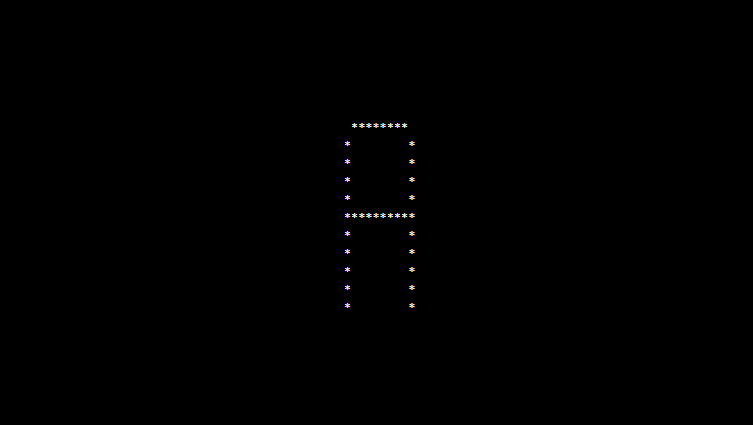# PHP program to print alphabet pattern A

In this article write a PHP program to print alphabet pattern A. This Program first takes the numbers of rows and then prints pattern using nested for loops.

## PHP program to print alphabet pattern A

```<?php
echo "<pre>";
for (\$row = 0; \$row <= 10; \$row++) {
for (\$column = 0; \$column <= 10; \$column++) {
if (((\$column == 1 or \$column == 10) and \$row != 0) or ((\$row == 0 or \$row == 5) and (\$column > 1 and \$column < 10))) {
echo "*";
} else {
echo "&nbsp;";
}
}
echo "<br>";
}
echo "</pre>";
?>
```

### Output: